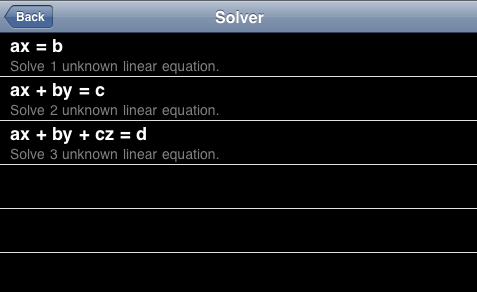# Solver## How to use Solver

You can solve three kinds of linear equations in this version. These use is the same like the followings.
1. Select equation in the equation list.
2. Input all cofficients.
3. Push Solve button, then the answer will be shown.
4. If you want to reset all cofficients, push Reset button.